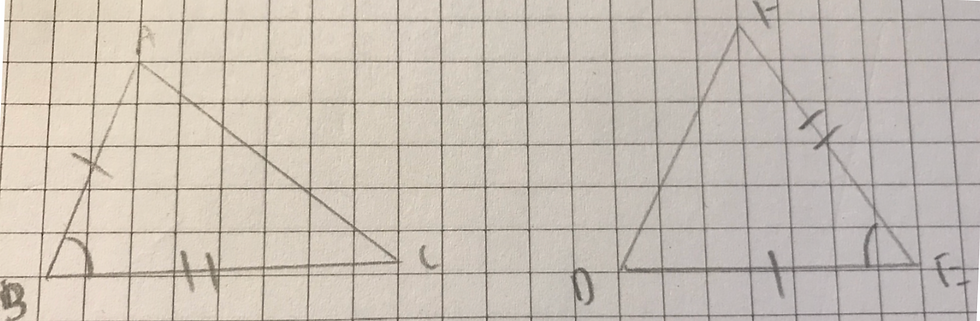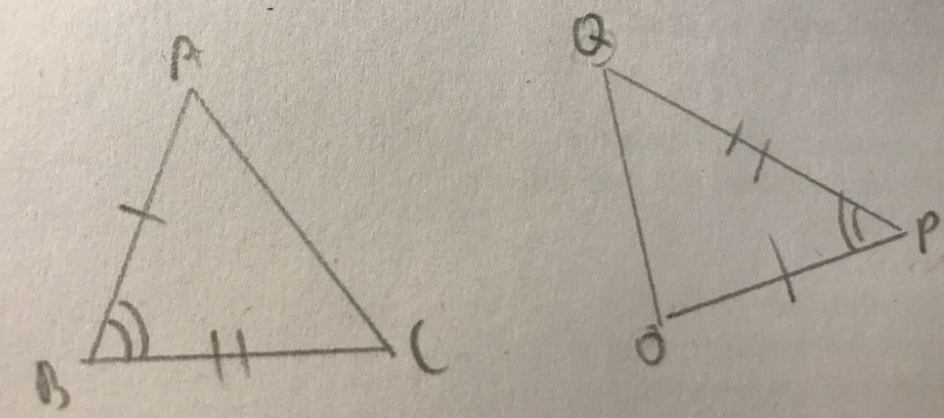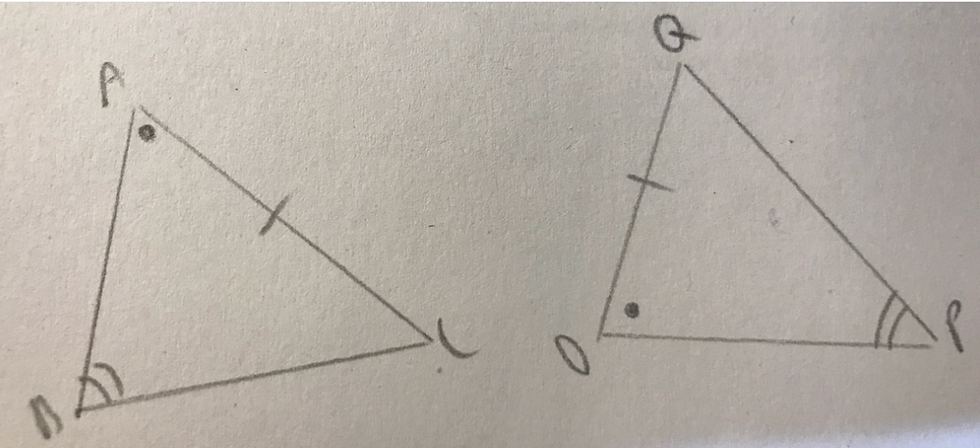top of pageSearch

# Basic Introduction to Triangle Congruence in Geometry

By Tara Li

Edited by Emma Davis

Triangles are congruent when they have exactly the same three sides, and exactly the same three angles. We represent congruence by using the symbol (≅).

### What is Congruence?

Congruence appears in all areas of our lives, in the same way geometric shapes form and structure our environments. For example, each A4 piece of paper you put in the printer has the exact same dimensions as the next. If you flip or turn the papers, they will still be identical to each other. In this instance, the pieces of paper are congruent.

When a piece of A4 paper is cut diagonally, two triangles will be formed. If another A4 page is cut in the same way, two identical triangles will be formed as well. Since the original A4 sheets of paper were congruent, the triangles produced in this case are also congruent (they are cut in the same way), as they have the same sides and angles.

### Conditions of Congruence in Triangles:

There are 5 primary conditions used in the topic of triangle congruence:

##### 1. SSS (Side-Side-Side)Two triangles are congruent, or equal when the 3 sides of a triangle are equal to the corresponding sides of the other triangle.

##### 2. SAS (Side-Angle-Side)Two triangles are congruent when 2 sides and the angle included between those sides of 1 triangle are equal to the corresponding sides and included angle of the other triangle. The “included angle” is the angle situated between two sides of a triangle.

##### 3. AAS (Angle-Angle-Side)Two triangles are congruent if any 2 angles and the non-included side of one triangle are equal to the corresponding angles and the non-included side of the other triangle. The non-included side refers to any side that is not the one in between the two angles mentioned.

##### 4. ASA (Angle-Side-Angle)Two triangles are congruent if two angles and the side included between the angles of one triangle are equal to the corresponding two angles and a side included between the angles of the second triangle.

##### 5. RHS (Hypotenuse-Side: Used for 2 Congruent Right-Angled Triangles ONLY)Two triangles are congruent if the hypotenuse and side of one right-angled triangle are equal to the hypotenuse and the corresponding side of another right-angled triangle.For example, you can say that the above two triangles are congruent because all 3 matching sides of each triangle are equal (SSS).

### Practical Application

Let’s take a look at how you would apply what you just learned in a proof! A proof is a structure used in order to show that a mathematical statement is true. In this case, we want to prove that triangles are congruent, therefore, we would use each condition above to help us construct a proof.

##### Structure of a triangle congruence proof:
1. Introduction of two triangles (in this case ABC and QRP)

2. Proofs (MUST have 3)

3. Ending condition for congruence (eg. SSS, SAS)

##### 1. Prove △ABC and △QRP are congruent.In △ABC and △QRP

AB = PQ (Given*)

AC = QR(Given)

BC = PR (Given)

∴△ABC ⩭△QRP (SSS)

In the first line, we have introduced the two triangles we are going to be proving congruent. After the introduction of the two triangles, you are going to start proving equal angles and/or equal sides. You simply do this by stating side __ = side __, and stating whether it is given or not. If it is not given, you must prove that the sides/angles you are talking about are equal beforehand.Each time you make a new statement in a proof, move to the next line. Every time a new section of the proof is being written, a line is left. In total, your congruence proof should have 5 lines, including the introduction and the conclusion. of working. After three lines of statements , you must close the proof with an ending reason. This is done in brackets after you write “∴△ __ ⩭△ __).

*Note: “Given” means that the equality of the sides, or any properties in general, are provided by the question.

Let’s try some more proofs. Try solving these ones on your own first before checking your answers below.

##### 2. Prove △ABC and △OPQ are congruent given the following diagrams.3. Prove △ABC and △OPQ are congruent given the following diagrams.4. Prove △ABC and △OPQ are congruent given the following diagrams.5. If AB = OP, AC = OQ and ABC and OPQ are both right angles, prove these two triangles are congruent.SOLUTION TO #2:

In △ABC and △OPQ

AB = OP (Given)

∠ABC = ∠OPQ (Given)

BC = PQ (Given)

∴△ABC ⩭△OPQ (SAS)

SOLUTION TO #3:

In △ABC and △OPQ

AC = OQ (Given)

∠ABC = ∠OPQ (Given)

∠CAB = ∠QOP (Given)

∴△ABC ⩭△OPQ (AAS)

SOLUTION TO #4:

In △ABC and △OPQ

AB = OP (Given)

∠ABC = ∠OPQ (Given)

∠CAB = ∠QOP (Given)

∴△ABC ⩭△OPQ (ASA)

SOLUTION TO #5:

In △ABC and △OPQ

AB = OP (Given)

∠ABC = ∠OPQ = 90° (Given)

AC = OQ (Given)

∴△ABC ⩭△OPQ (RHS)

Now that you have a basic understanding of triangle congruence, try applying your knowledge by looking for congruence in the world around you! Good luck!

#### Sources Cited:

https://www.cuemath.com/geometry/congruence-in-triangles/